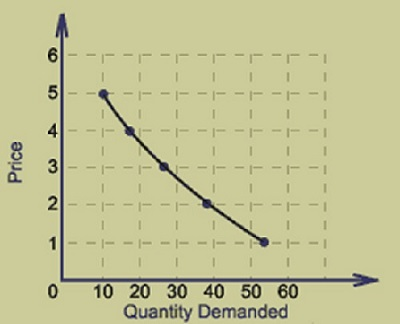# Consumer Behavior - Demand Analysis

## The DemandCurve

The quantity of a particular good or service that a consumer or group of consumers want to purchase at a given price is termed as demand. It is the consumer’s ability or willingness to buy a specific product.As shown in the figure, the demand curve is downward sloping which means the consumers will buy more when the price decreases and the same consumers will buy less when the price increases.

It is not only price, the demand for a good or a service is also influenced by other factors such as the price of substitute goods and complementary goods.

## Determinants of Demand

The key determinants that affect the demand function are as follows −

• Income − A rise in consumer’s income will tend to increase the demand curve (shift the demand curve to the right). A fall will tend to decrease the demand for normal goods.

• Consumer Preferences − Favorable change leads to an increase in demand, unfavorable change leads to a decrease in demand.

• Number of Buyers − More the number of buyers, more will be the demand. Fewer buyers lead to a decrease in demand.

• Substitute Goods (goods that can be used to replace each other) − The price of substitutes and demand for the other good are directly related. Example − If the price of coffee rises, the demand for tea will also rise.

• Complementary Goods (goods that can be used together) − The prices of complementary goods and their demand are inversely related. Example − if the price of printer increases, the demand for computer sheets will decrease.

## Demand Function

The demand function relates to the price and quantity. It shows how many units of a good will be purchased at different prices. At higher prices, less quantity will be purchased.

The graphical representation of the demand function has a negative (-ve) slope. The market demand function is calculated by totaling up all of the individual demand functions.

### Demand Function of an Individual

The individual demand function has a functional relationship between individual demand and the factors affecting individual demand.

It is expressed as −

Dx = f (Px, Pr, Y, T, F)

Where,

Dx = Commodity Demand x;

Px = Commodity x”s price;

Pr = Related Goods’ Price;

F = Expectation of Change in Price in future.

Y = Consumer’s Income;

T = Tastes and Preferences.

### Demand Function of Market

The market demand function has a functional relationship between market demand and the factors affecting market demand.

The market demand function can be expressed as −

Dx = f(Px, Pr, Y, T, F, PD, S, D)

Where,

Dx = Market demand of commodity x;

Px = Price of given commodity x;

Pr = Related Goods’ Price;

Y = Consumer’s Income;

T = Tastes and Preferences;

F = Expectation of Change in Price in future;

PD = Size and Composition and Size of population;

S = Season and Weather;

D = Income Distribution.